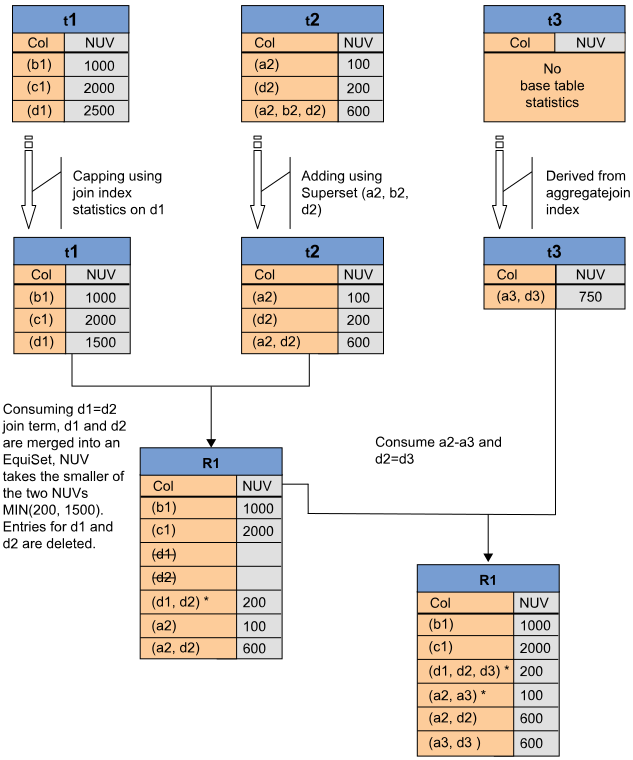# Derived Statistics | Optimizer Process | Teradata Vantage - 17.10 - Derived Statistics - Advanced SQL Engine - Teradata Database

## Teradata Vantage™ - SQL Request and Transaction Processing

Product
Release Number
17.10
Release Date
July 2021
Content Type
Programming Reference
User Guide
Publication ID
B035-1142-171K
Language
English (United States)

The initial cardinality estimates based on interval histogram statistics, from which the Optimizer begins the process of query optimization, are adjusted dynamically throughout the optimization process to gain increasing accuracy by applying information derived from various database constraints, query predicates, and hash and join indexes.

Because of the way the system calculates these statistics, they are referred to as derived statistics. Derived statistics enable the Optimizer to achieve the same end result of dynamically reoptimizing a request in mid-query when it has been determined to have a suboptimal plan without having to reoptimize the entire query from the beginning.

## Definition of Derived Statistics

Derived statistics are snapshots, derived from base table interval histogram statistics, that the Optimizer initializes and transforms while it optimizes a query. They are cardinality estimates that are transformed from various constraint sources, including query predicates, CHECK and referential integrity constraints, and hash and join indexes, and then adjusted dynamically at each stage of the query optimization process. In other words, derived statistics represent the demographics of column values after applying query predicates and demographic information derived from other sources.

Optimizing queries based on information derived from integrity constraints falls into the general category of semantic query optimization, which was initially developed in the field of deductive databases. Note that semantic query optimization methods can also add or subtract derived constraints from a request in order to better optimize it.

For example, the Join Planner needs to know the demographics of join columns after applying single-table predicates or after making a join. All this information is derived and propagated throughout query optimization using the derived statistics infrastructure, and it is used consistently in all cost and estimation formulas.

The derived statistics framework also employs several techniques for dealing with stale statistics, including comparing cardinality estimates obtained from a dynamic AMP sample with the statistics stored in the relevant interval histogram, and bidirectional inheritance of statistics between a base table and its supporting indexes, using whichever set has the more recent collection timestamp. See Statistical Inheritance by Hash and Join Indexes.

Bidirectional inheritance is the term used to describe how base tables and their underlying indexes are able to inherit and use existing statistics from one another when either database object in an index-table pair has no existing interval histogram statistics.

If both database objects have existing interval histogram statistics, the Optimizer assumes that the more recently collected statistics are the more accurate, so it uses the set with the more recent collection timestamp.

## Derived Statistics Flow

The following table and join index definitions are used for the query that illustrates the flow of derived statistics usage by the Optimizer to generate more accurate cardinality estimates:

```CREATE TABLE t2 (
a2 INTEGER PRIMARY KEY,
b2 INTEGER,
c2 CHARACTER(1) CHECK (c2 IN ('M', 'F')),
d2 DATE);

CREATE TABLE t3 (
a3 INTEGER,
b3 INTEGER,
c3 CHARACTER(5),
d3 INTEGER);

CREATE JOIN INDEX ji_t1 AS
SELECT a1, d1
FROM t1
WHERE b1 > 10

CREATE JOIN INDEX aji_t3 AS
SELECT a3, d3, COUNT(*)
FROM t3
WHERE b3 < 100
GROUP BY 1,2);```

Given these table and join index definitions, the flow chart that follows later in this topic shows how the Optimizer uses derived statistics to more accurately estimate cardinalities for the following query:

```SELECT *
FROM t1, t2, t3
WHERE b1 > 10
AND   b3 < 50
AND   d1 = d2
AND   a2 = a3
AND   d2 = d3;```

The stages of deriving various statistics for this query are as follows:

1. Refine and derive base table statistics using join index statistics.
2. Join tables t1 and t2, consuming the term d1=d2 and producing the interim join relation R1.

d1 and d2 are then merged into an EquiSet (see Using Join Predicate Redundancy to Derive Column Demographics After Each Binary Join), which takes the smaller of the 2 unique values cardinalities as MIN(200,1500), or 200.

The entries for d1 and d2 are then removed from the derived statistics set.

3. Join table t3 with the interim join relation R1, consuming the terms a2=a3 and d2=d3 and producing the following set of derived statistics cardinalities for join relation R2.
R2
Column Set Number of Unique Values
(b1)                  1000
(c1)                  2000
(d1, d2, d3)                    200
(a2, a3)                    100
(a2, d2)                    600
(a3, d3)                    600